## Voltage Magnification in Series Resonance:

Voltage Magnification in Series Resonance – If we assume that the voltage applied to the series RLC circuit is V, and the current at resonance is I, then the voltage across L is VL = IXL = (V/R) ωrL.

Similarly, the voltage across C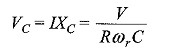Since ,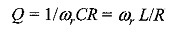where ωr is the frequency at resonance.

Therefore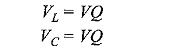The ratio of voltage across either L or C to the voltage applied at resonance can be defined as magnification.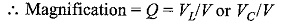### The Quality Factor (Q) and its Effect on Bandwidth:

The quality factor, Q, is the ratio of the reactive power in the inductor or capacitor to the true power in the resistance in series with the coil or capacitor.

The quality factorIn an inductor, the max energy stored is given by LI2/2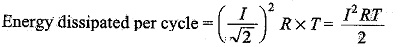Quality factor of the coil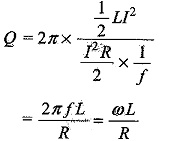Similarly, in a capacitor, the max energy stored is given by CV2/2

The energy dissipated per cycle = (I/√2)2 R x T

The quality factor of the capacitance circuit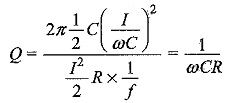In series circuits, the quality factor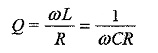We have already discussed the relation between bandwidth and quality factor, which is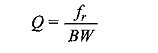A higher value of circuit Q results in a smaller bandwidth. A lower value of Q causes a larger bandwidth.

Scroll to Top